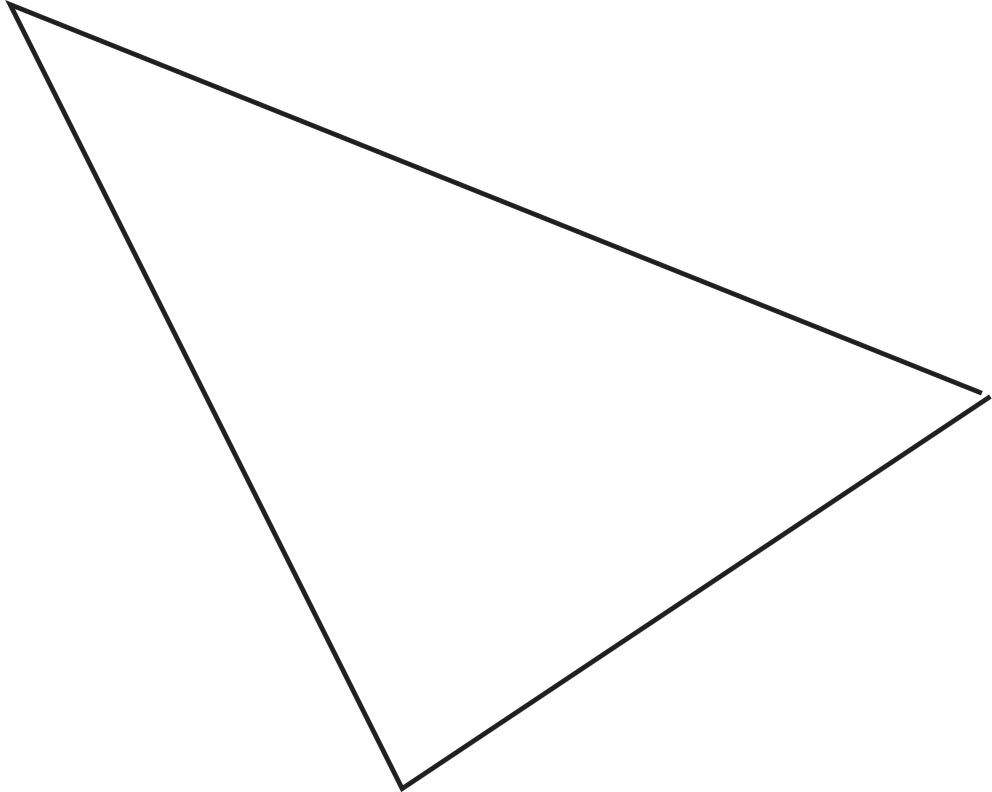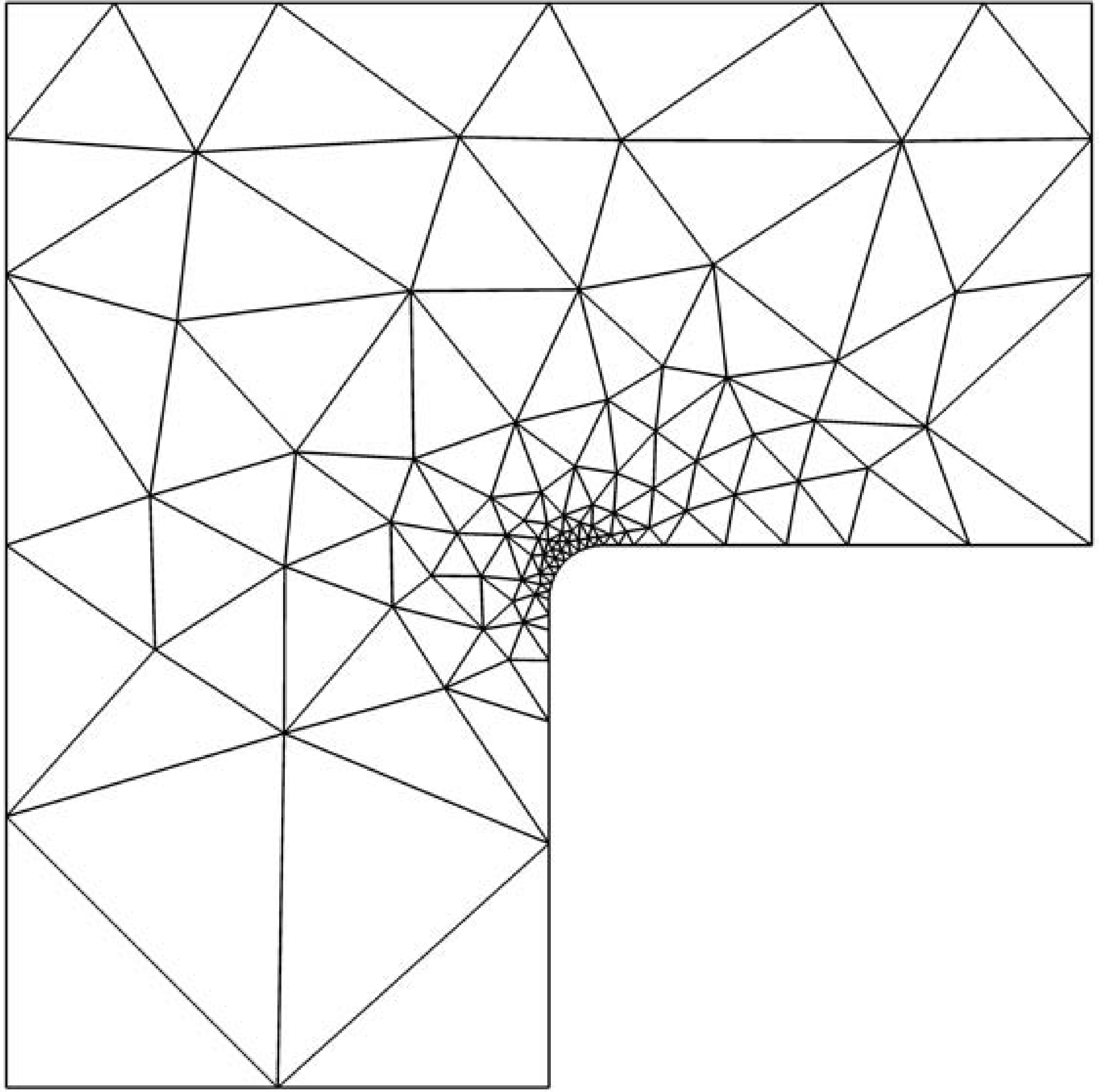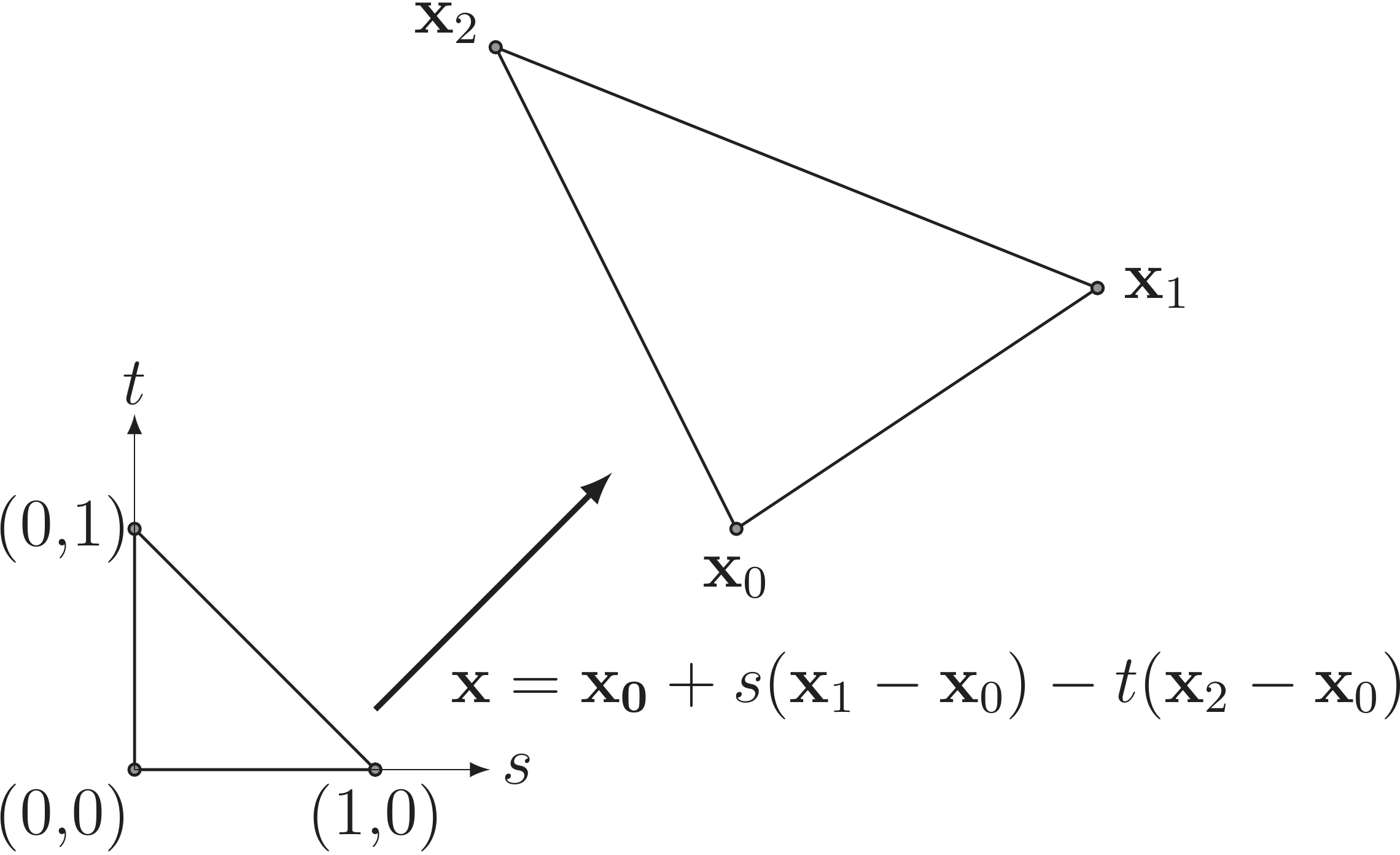Previous: 3.6 Void Evolution    Top: Home    Next: 4.2 Implementation in FEDOS

### 4 Numerical Implementation

Finding the analytical solution for partial differential equations in three dimensions is in general only for quite simple problems regardinggeometries,boundary conditions,boundaries between domains with different material behavior, andmaterial behavior
possible. General TCAD simulations are to complicated and numerical methods have to be used. Numerical methods produce approximations of the solutions. One of the methods mainly used and widespread for Partial Differential Equations (PDEs) in engineering is the Finite Element Method (FEM). Due to the availability of powerful computers since the midcentury this method got practicable. Since this method was used for the simulations in this work, the basics will be described in this, followed by a discussion of the implementation of the EM model in the two software packages used.

#### 4.1  Finite Element Method

The finite element method is a common approach to solve PDEs . Thereby the simulation region is filled with a mesh and the solution is approximated by a linear combination of weighted base functions, which is called the Ritz-Galerkin-approximation . This approach enables the software to approximate the solution by solving a system of linear equations representable by matrix equations.

A second order PDE can generally be written asforWithout loss of generality it can be assumed that, which easily can be achieved due to the commutativity of the differentiation order.
Definition 1. The differential equation 4.1 is elliptic in, iff all eigenvalues of the matrixhave the same sign and are not equal to zero.
Definition 2. The differential equation 4.1 is elliptic (in), iff it is elliptic in (almost) allFor the further descriptionwill be set to three, as the simulations are carried out in the three-dimensional real space and the time derivatives are realized by time stepping to reduce the problem order by solving it iteratively approximated by a finite differences schema. Furthermore, the material parameters in EM are rotationally symmetric leading to the following simplified form of the differential equations, where the symbolsandare used for the nabla and the Laplace operator, respectively.##### 4.1.1  Weak Formulation of PDEs

In order to derive the weak formulation of (4.2) one first has to multiply (4.2) by a test functionand then integrate the result over the domaingivingFor FEM based simulations only gradients of the solutionand of the test functionoccur in the integral, allowing the usage of solution functions with lower smoothness. This makes the employment of Green’s first identity necessary.
Theorem 1. Divergence theorem: Ifis a vector field andis an outward pointing normal vector of the surfacesurrounding the volume, then the volume integral of the divergence ofcan be converted to the flux integral through the surface byApplying Theorem 1 to a vector field defined byleads to Green’s first identity .
Theorem 2. Green’s first identityEmploying Theorem 2 to (4.3) results in the so called weak formulation of the problem, which is given bywhere the first part is a surface integral and the second integral is carried out over the whole volume. For the further discussion it is assumed thatis a material parameter being piecewise constant and therefore not depending on the position. For example in the drift-diffusion equation of the vacancies it is proportional to the diffusion coefficient (c.f. Section 3.2).

##### 4.1.2  Meshing of the Simulation Domains

Before the actual solving of the PDE can be started, the simulation domain has to be meshed. The meshing procedure subdivides the simulation domain into many small elements, described by nodes, edges, and faces. The generated mesh can either be structured by simple subdivision rules or unstructured for arbitrary domains. Typical shapes used for FEM are triangles (cf. Figure 4.) and quadrilaterals (cf. Figure 4.) for two-dimensional (2D) domains and tetrahedrons (cf. Figure 4.) and hexahedrons (cf. Figure 4.) in 3D simulation domains . Figure 4.2 shows an example triangular mesh for anshaped 2D domain. The mesh is refined at locations of higher interest and smaller geometrical features. In this example this is the fillet, as the boundary is a quarter of a circle.Figure 4.1.:  Example meshing elements for 2D and 3D domains.

Based on the meshing and its element type a simple reference element can be chosen establishing a mapping. For triangular meshing this mapping is shown in Figure 4.3. Due to this mapping the integration of every element can be formulated as an integrationFigure 4.2.:  An example mesh for an shaped structure with a fillet.

over the reference element by employing the determinantof the Jacobian matrix. If the mapping can be written as a linear combination of the vectors spanning the element, where the origin of the element is one of the corner coordinates, the integral melts down to the volume of the original element times an integral not depending on the original element giving the possibility to precompute the integrals of (4.6). For triangular meshing elements (cf. Figure 4.3) the transformation of the integral over an element and the reference element is given byImportant for the transformation is the preservation of the orientation. Choosing for this meshing a test function spaceand, therefore, a solution space leads to the Ritz-Galerkin-approximation .Figure 4.3.:  Mapping between an arbitrary triangle and the reference triangle for 2D triangular meshing with the transformation law.
##### 4.1.3  Ritz-Galerkin-Approximation

Letbe(real) Hilbert space anda continuous bilinear form andbounded linear functional on. To solve a problem, given byapproximately, the infinite spaceis substituted by the finite space. The Ritz-Galerkin-Approximationis defined as the solution ofThis problem is finite-dimensional and withbeing a complete basis ofthe solution can be written by the linear combination(4.9) has to be valid for alland to solve the problem it is enough to use only the basis functionstransforming the problem toand by substitutingby (4.10) a system oflinear equationswithunknowns is obtained. By defining the matrixand the vectorsandthe system can be written in matrix notationand reduces the implementation to the calculation of the solution of a system of linear equations.Figure 4.4.:  The hat test function for a single node located in the middle.

A typical test function used for the weak formulation (4.6) is the so called hat function shown in Figure 4.4 . It is defined to be 1 at the meshing point considered and decreases linearly in direction of the neighboring meshing points to zero. For a single triangle the function is defined byFor the reference triangle the coordinates of the points are given byand a general coordinate byresulting in the hat functionThe same procedure can be applied to the other two meshing points of this triangle leading to the functionsandThe weak formulation (4.6) can now be transformed in a summation of integrals over each of thetriangles, leading toand by applying the approximation of all functions as a linear combination of the hat functions given byformesh points it followsAs this result has to be valid for every functionit is enough to choose only one hat function at a time.The primed coordinate system is the coordinate system of the reference system and allows the calculation of every integral in advance. This leads to the matrix representationThe elements of matrixare defined byand of matrixbyand, andis represented by the vectors, and, respectively. Theare joined into the matrixThese are sparse matrices as only those integrals in (4.26), where theandare edges of the triangle that is integrated over are not zero.

Previous: 3.6 Void Evolution    Top: Home    Next: 4.2 Implementation in FEDOS